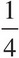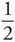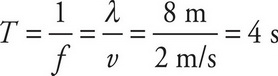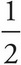# AP Physics 1 Question 257: Answer and Explanation

### Test Information

Question: 257

3. A transverse wave on a long horizontal rope with a wavelength of 8 m travels at 2 m/s. At t = 0, a particular point on the rope has a vertical displacement of +A, where A is the amplitude of the wave. At what time will the vertical displacement of this same point on the rope be -A ?

• A. t =s
• B. t =s
• C. t = 2 s
• D. t = 4 sso the desired time is(4 s) = 2 s.